# Catch up on the latest AI articles# Physically Embedded Planning: A New Challenge For Reinforcement Learning! Can Robots Solve Symbolic Games?

3 main points
✔️ Proposed RL environment on new physically embedded planning problems
✔️ Proposed a method of a benchmark for solving problems

✔️ An expert planner allows robots to solve problems, but the efficiency is currently poor.

Subjects: Artificial Intelligence (cs.AI); Machine Learning (cs.LG)
Paper
Official Code COMM Code

## Introduction

Here's a paper that mentions a new challenge in reinforcement learning that came out of DeepMind. The first thing that comes to mind when you think of famous reinforcement learning applications is Alpha Go from DeepMind. Alpha Go allows you to learn the best possible placement in Go and is actually used by professionals You may remember that you beat a Go player. However, in a game like Alpha Go, even if you can find the best placement, it's the people who actually move the games. In the actual matchup, a human player places a go in that spot based on the layout output from Alpha Go. Can a robot, not a person, grab a go and place it exactly where it wants to be placed? Looking at the diagram below, we can see that reinforcement learning can solve for each of the three symbols in a game (white is 0, black is 1, etc.) using symbols to represent the state of the game, but can it be done correctly in a situation where the game is controlled by a robot? These are called Physically Embedded planning problems. This is considered to be difficult for reinforcement learning because you have to take into account perception, reasoning, and motor control over a long horizon. DeepMind provides an environment that provides these kinds of problems, so if you are interested, please check it out. In this article, I will explain these issues in more detail and then I will end with some brief experimental results shown in the paper.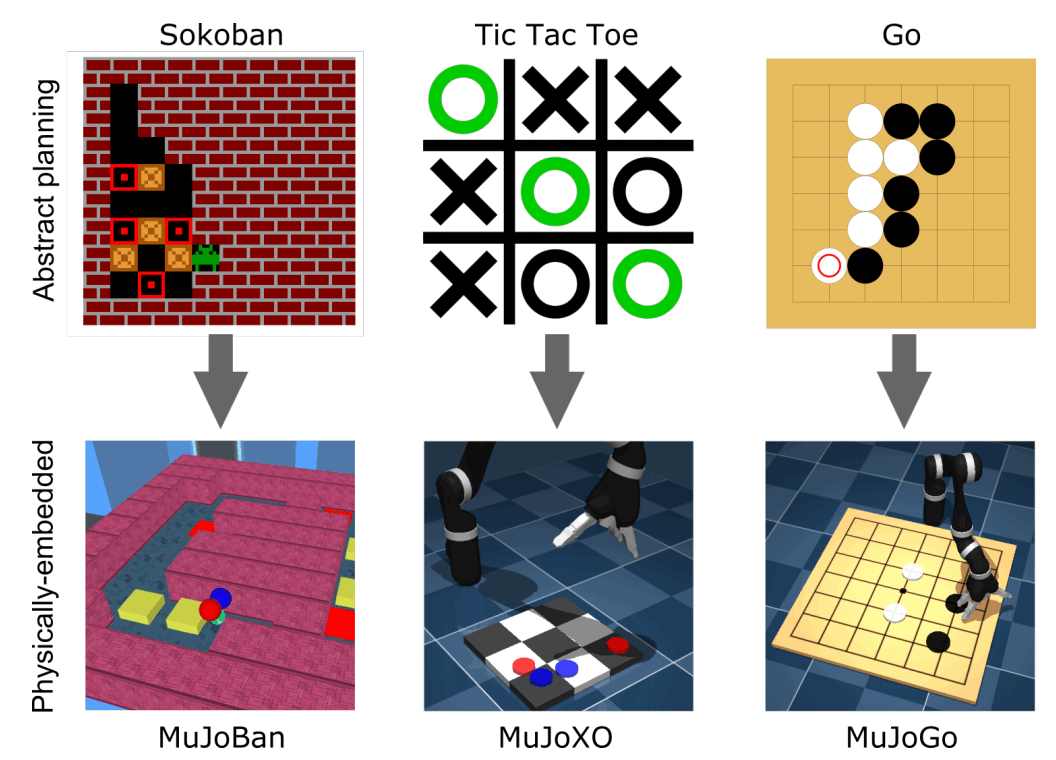## Background

This chapter introduces the challenges of motor control and why it is so difficult to reason in a long-horizon task.

### Challenges in Motor Control

There is a wide range of research on robot control. For example, many studies have focused on the dexterity of robots and long-horizon tasks. However, the combination of the complexity of operating the robotic arm and the long-horizon task has not been studied much. Also, such problems are particularly difficult with high-dimensional inputs, such as images, and the problem is particularly difficult when the action space is continuous. Basically, the problem can be solved from image input to abstract information (grabbing an object, placing an object, etc.) ) can be extracted to solve the problem, but the current difficulty in extracting this information makes motor control difficult.

### The rationale in long-horizon tasks

The long-horizon task is very difficult, e.g. in Go, when a decision has a large impact on the future, and the positive reward for an action taken at that time comes very late, it is important to reason about the action and the impact it had on the future becomes very difficult. Thus, learning to reason at a high level and to control a robot based on that reasoning is a very difficult problem. Another common problem in reinforcement learning is hard exploration, which is especially noticeable in long-horizon tasks. This means that when you have to find a rare strategy that will reward you with a variety of strategies, it is very unlikely that you will find that strategy. One way to solve this hard exploration would be to define the reward in more detail and give information to the agent. We can also consider methods such as curricular learning, which gradually makes the problem harder to solve, to learning by demonstrations from expert agents, and intrinsic rewards, where the agent is rewarded with an unknown There are ways to encourage people to explore newer states, such as by giving them a reward when they visit a state.

## Environment

An example of the environment prepared by DeepMind is shown in the diagram above, which includes a Go problem and more. In this chapter, we'll go into more detail about each of these games.

### MuJoBan

MuJoBan is an environment based on the MuJoCo simulation, which is based on Sokoban, a single-player puzzle-solving game. The left side of these two environments is the top view and the right side is the player's view. In this puzzle, the agent can push the yellow object, but cannot pull it. Therefore, if you don't plan to push the object, you won't be able to pull the object after pushing it, and you won't be able to solve the puzzle. Therefore, planning is very important. In this provided environment, the environment looks different, the difficulty of the maze, and the size of the maze.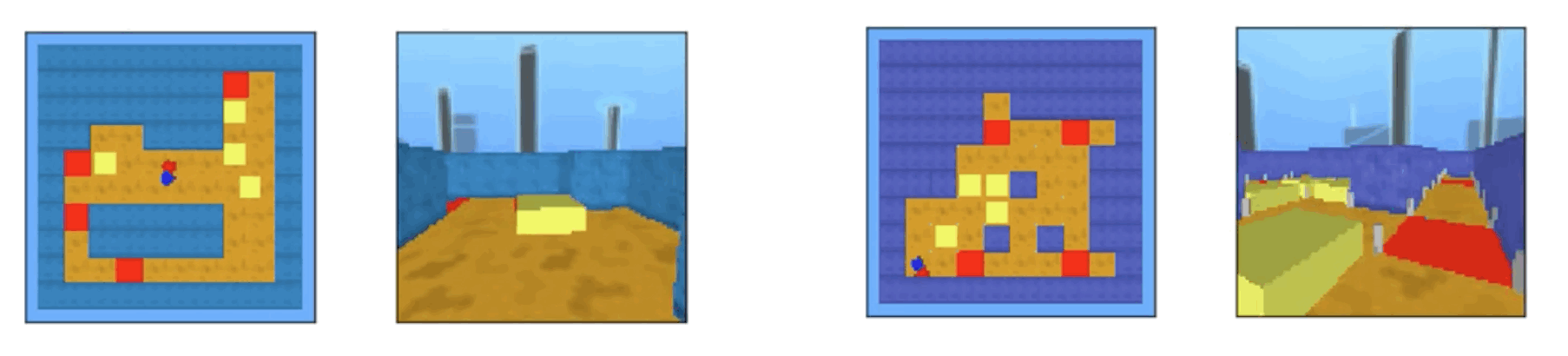The agent in this environment is a 2DoF, which allows you to move objects by rotating your body relative to the object. Three types of observations are available: joint torque, velocity, and position of the object, the environment as seen from above, and the agent's perspective. The agent has ears, blue on the left and red on the right, to show the agent's state when viewed from above. If the agents are able to solve the problem by moving each object onto the red pad, they are rewarded at the end of the episode with a $10$ reward and $1$ for each object placed on the red pad, and You are rewarded $1-1$ if you fail to solve the puzzle. Basically, some simple problems can be solved by moving the agent about 200 times, while others are more difficult and require about 900 moves to solve.

Also, when Sokoban is a game where the agent has to push an object to solve it, the game may not reflect all the rules correctly. For example, the agent cannot push an object in the corner of the maze because he cannot pull it. Therefore, in this environment, the rules are a little different, and if the agent moves like rubbing against an object, the object is separated from the corner by the friction and can be moved. There are other constraints, such as the inability to move objects at an angle.

### MuJoXO

MujoXO is an implementation of the tic-tac-toe game in the physics engine, where the robot arm touches the correct pad on the board and the agent's colored pieces appear on the pad. Then, after the piece is placed, the opponent's piece is placed using an abstract planner. The position of the pad has been noisier in light of the real-world situation. Then, at the beginning of the episode and after each move, the robot's position on the board changes randomly. To represent the difficulty of the various problems, the opponent's moves are given in a way that uses a \\courtesy$-greedy, with a probability of a random move in \courtesy$ and an optimal move in the remaining probability.

The reward is $0$ for the duration of the game, $1$ if you win the game, and $0.5$ for a tie; Observation is given for Joint angle, velocity, torque, end-effector coordinates, and coordinates about the board. Other image inputs are also available, as shown below. Using the trained agents, the game can be completed in about 100 steps.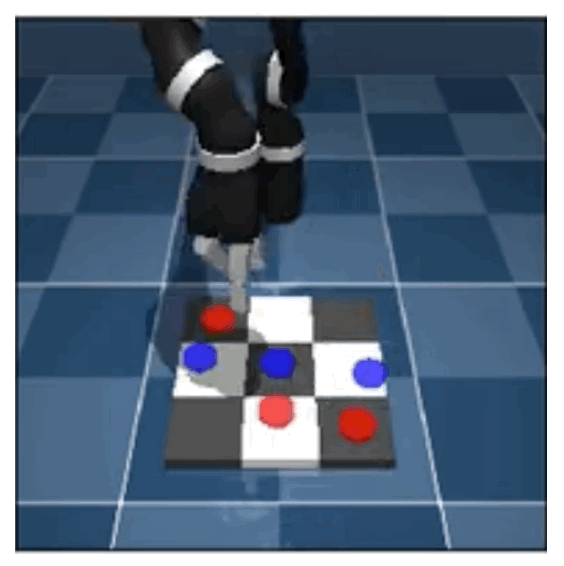### MuJoGo

Lastly, I'd like to introduce MuJoGo, an implementation of Go in MuJoCo. In this environment, there is a 7x7 grid with pads at the intersection of each grid, and the robot's end-effector touches the intersection to place a go. Also, you can pass the game by touching the spaces on the right and left sides of the grid as shown in the diagram below. You are given a time limit to solve this problem, and you lose if you exceed the time limit. With an efficient agent, it takes about 50 moves to finish the game. Your opponent's moves are determined by the GNU Program, a go program. The opponent's strength is determined by using $\epsilon$-greedy as well as MuJoXO.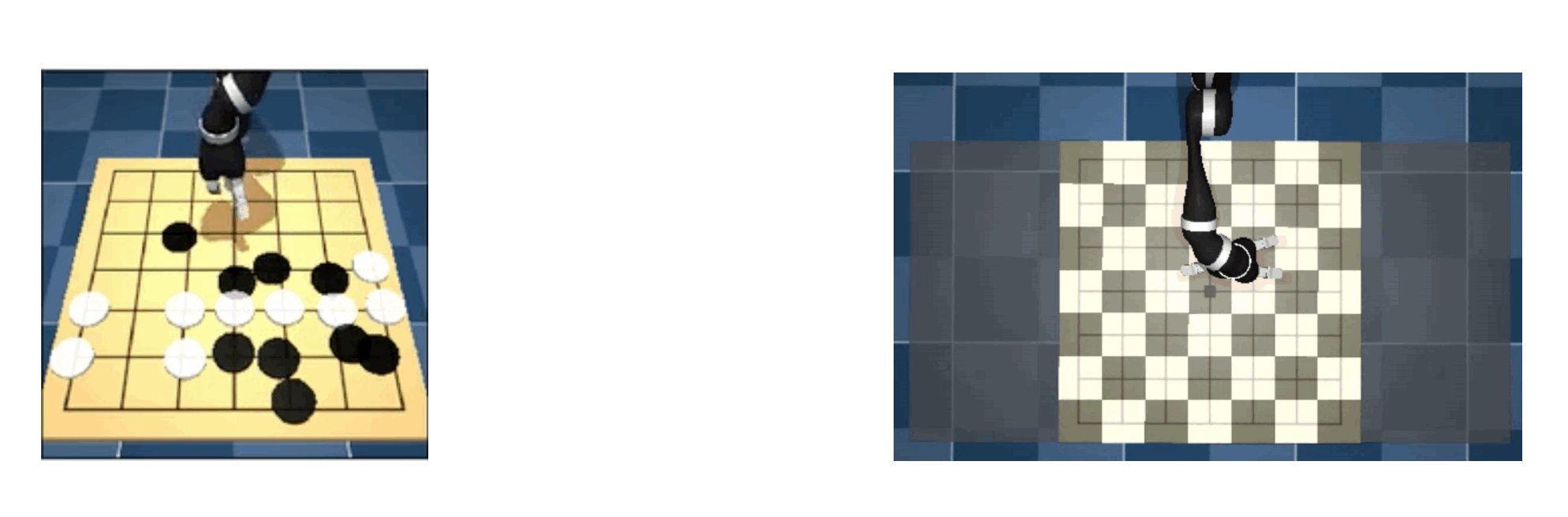## Experiment

In this paper, we are experimenting with this environment as a baseline for future use in research; although we have basically no success in learning using State-of-the-art RL methods, we have been able to give the actor-critic information about the expert planner We have found that the results are somewhat better on all tasks in This shows that by defining the reward function more finely, information about high-level strategies is transferred to the RL agents to learn the plan. Now, we investigated how much abstract information about the task, e.g., state, dynamics, or solutions, must be given in order to see what shortcomings the usual RL methods have in this environment. Specifically, the experiment was conducted under the following three conditions

1. provide information about State, Dynamics, and Solution (Expert Planner condition)

2. give information on State and Dynamics, but not on Solution (Random Planner condition)

Give minimal or no abstract information (Vanilla agent condition)

### Agent Structure

The structure of the Agent is as follows and an actor-critic network is used. The training is trained using the distributed IMPALA actor-critic algorithm. The structure below differs in two ways from the structure of the other methods used in continuous control in two ways. One is that it contains an expert planner, which maps the abstract states of the ground truth to the abstract states of the target and the actions of the game to get there. Since these states are difficult to infer from information such as images, and this abstract information is sufficient to solve the problem, we consider incorporating this information to investigate what role these states play in the problem in particular.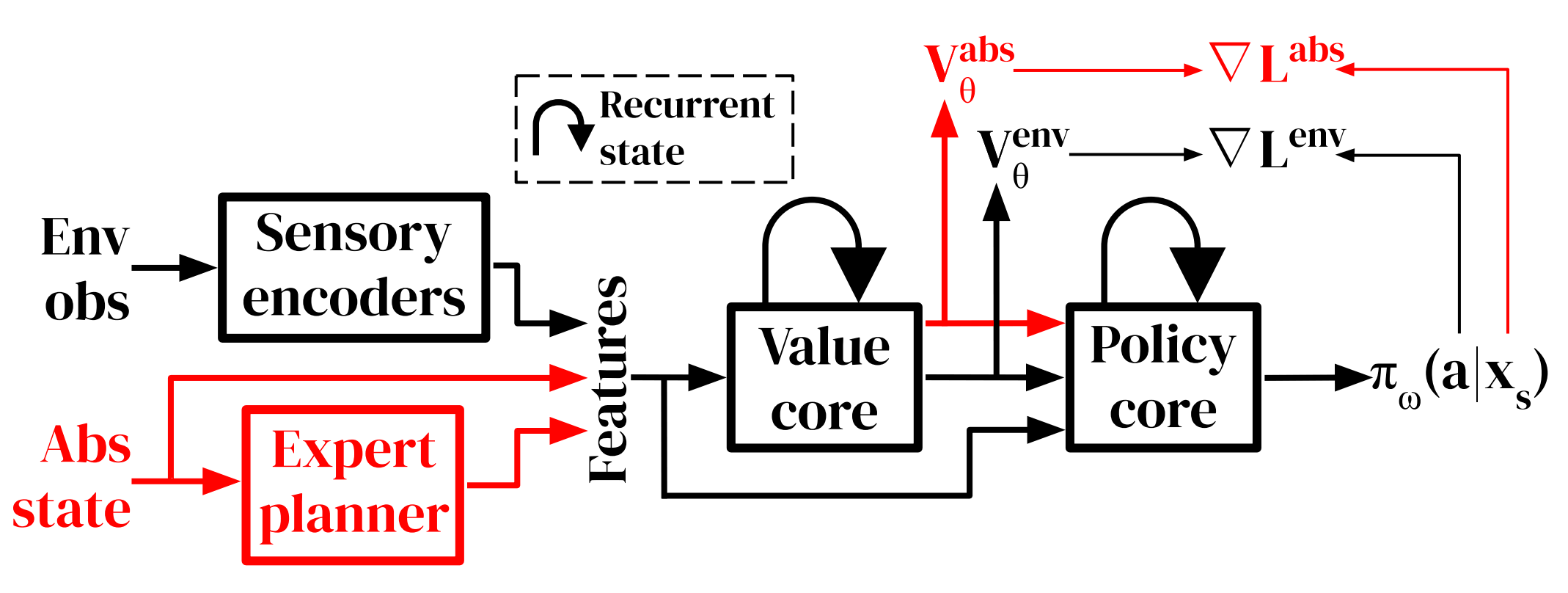Another different part is the addition of an auxiliary task to follow the instructions of the expert in abstract states. This means that the agent will receive a reward when it is able to visit a state-space transition given by the expert. This auxiliary task is shorter than the original length of the task and has a fixed time limit. The auxiliary task is reset when the agent solves the auxiliary task, or when the time limit is exceeded. By giving this task to the agent, we can provide the agent with information about the abstract state space, and then we can tell the agent how the low-level robot's movement affects the state-space.

In this method using actor-criticism, we have to predict the value. In our method, we use two different neural networks to train the value for the main task and the value for the auxiliary task separately. The policy gradient equation needed for the final agent update is given by

$$\nabla \mathcal{L}^{e n v}=\mathbb{E}_{x_{s}, a_{s}}\left[\rho_{s} \nabla_{\omega} \log \pi_{\omega}\left(a_{s} \mid x_{s}\right)\left(r_{t}^{e n v}+\gamma^{e n v} v_{s+1}^{e n v}-V_{\theta}^{e n v}\left(x_{s}\right)\right)\right]$$

$$\nabla \mathcal{L}^{a b s}=\mathbb{E}_{x_{s}, a_{s}}\left[\rho_{s} \nabla_{\omega} \log \pi_{\omega}\left(a_{s} \mid x_{s}\right)\left(r_{t}^{a b s}+\gamma^{a b s} v_{s+1}^{a b s}-V_{\theta}^{a b s}\left(x_{s}\right)\right)\right]$$

The first expression relates to the main task and the second to the auxiliary task. $\gamma$ is the discount factor, $x_{t}$ is the state, and $v_{s+1}$ is a value target. $r_{t}$ represents compensation, $a_{s}$ represents motor action, $\pi_{\omega}$ represents policy, and $\rho_{s}$ represents importance sampling weight.

In the expert planner method, the current abstract state and the target abstract state predicted by the expert planner are used as value and policy inputs. Other inputs are given by the agent, such as image information, and velocity, touch, position and acceleration, depending on the task.

## Results

The following graph shows the results of the experiment on MuJoBan, which shows how difficult it is to train with the usual RL methods, but on the contrary, it is more accurate when using a planner. The "With grid pegs" is more like the original Sokoban, as you can't push objects at an angle, making it more difficult as a task. As you can see in the graph below, the success rate for "With grid pegs" is higher with the expert planner than with the random planner, while the success rate for "Without grid pegs" is higher with the random planner. It makes sense. This may be due to the fact that in the case of "Without grid pegs", the planner and the actual problem do not match up well with each other due to the flexibility of the "Without grid pegs", which may make the information in the expert planner less useful The "with grid pegs" approach is much closer to the problem to be solved. On the other hand, "with grid pegs" is much closer to the problem to be solved, and the expert planner information is more useful because it assumes that the predicted subgoal is essentially on the best path, and thus the success rate is higher than with the random planner results It is believed that this is the case.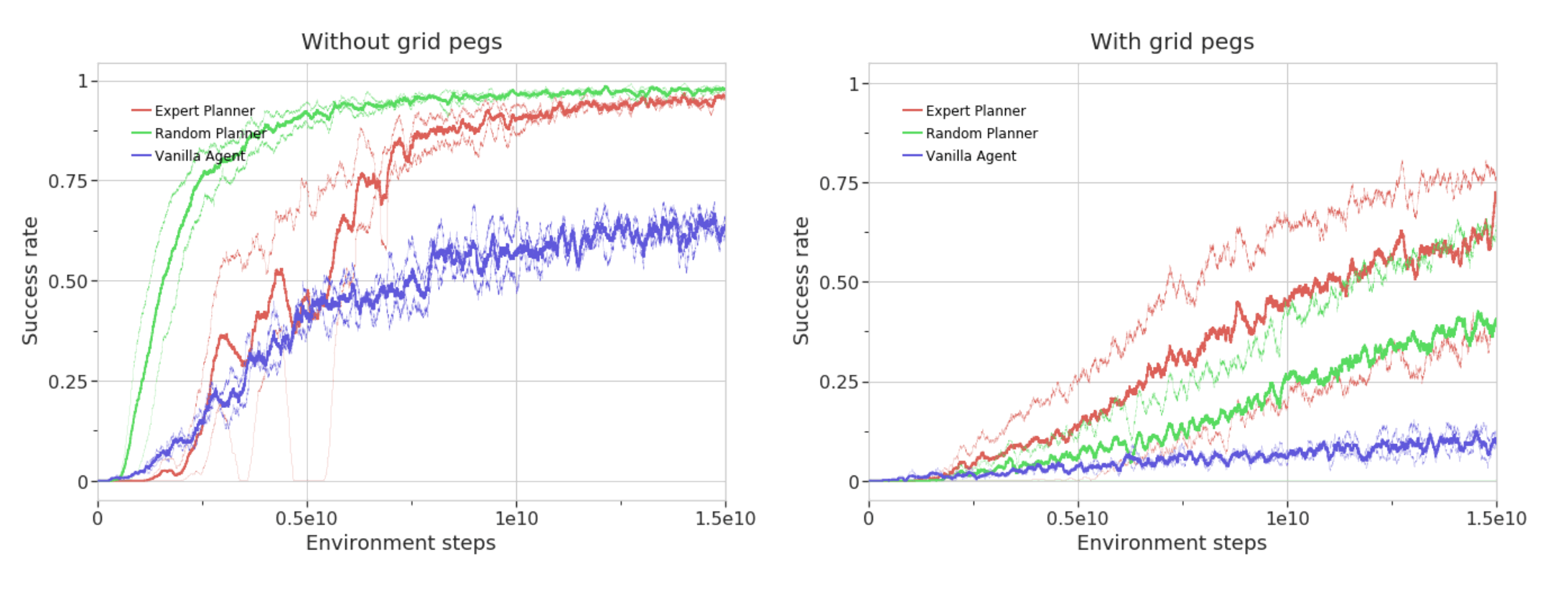Finally, the results for MuJoXO and MuJoGo, although MuJoXO has less branching than the other tasks and planning is relatively easy, it nevertheless requires a lot more data to be trained, even with an expert planner. The planner is relatively easy to plan, but even with an expert planner, it requires a lot of data to learn. The expert planner, on the other hand, has no mismatch between the original game and the physics simulation and allows the expert planner to choose the best strategy, but nevertheless provides a reliable way for the agent to win the game. The relatively low value of the acerage return shows that this is not enough to find

As for MuJoGo, the game itself is more difficult to learn, due to its length and greater complexity. Regular RL is not learning at all because it is so difficult to explore; agents using the expert planner were able to eventually beat their opponents, but the learning was very inefficient. In our experiments, we have to play about 4M games before we reach a 60% win rate. Thus, it is basically difficult to solve the problem in normal RL, but by using expert planner, we have found that the learning is not efficient, but we can still solve the problem.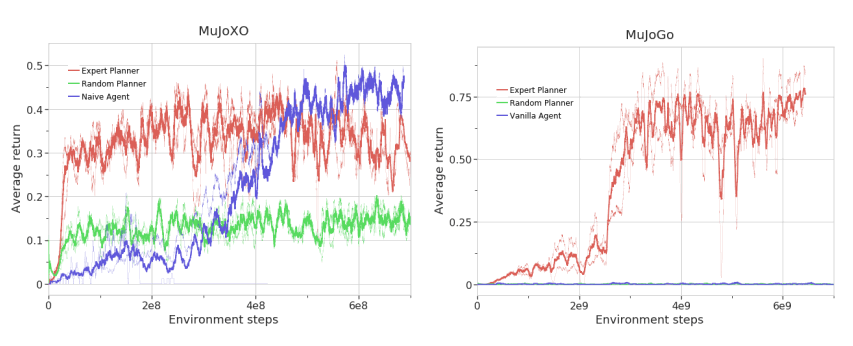## Summary

In this article, I have introduced a new challenge in reinforcement learning. The games presented in this article are important for abstract reasoning and the associated motor control, and I think they suggest a very useful environment for research in this direction using reinforcement learning. Humans take cues from expert demonstrations and other games in a variety of ways, for example. With this in mind, I am looking forward to seeing what new RL algorithms can be used to solve more efficiently in this new environment in the future.

If you have any suggestions for improvement of the content of the article,